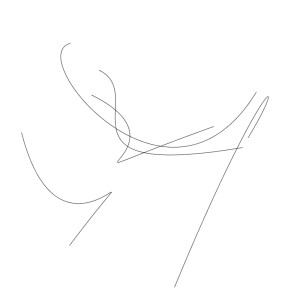# Contains the tag: 2014## Void Shuffle (int[] array)

Series of 1 minute videos arranged according to the shuffle function of the source its being played from. The series contain snippets and information recorded during the making of the piece. Shots of the studio, the travel to the studio etc. are mixed up with tutorial videos on shuffle and random code. In the midst […]

More...## 800x800pixeldrawing-####.tif

void setup() { size(800, 800); background(255); } void draw(){} void mousePressed(){ int x = (width); int y = (height); noFill(); bezier(random(x), random(y), random(x), random(y),random(x), random(y),random(x), random(y)); bezier(random(x), random(y), random(x), random(y),random(x), random(y),random(x), random(y)); bezier(random(x), random(y), random(x), random(y),random(x), random(y),random(x), random(y)); bezier(random(x), random(y), random(x), random(y),random(x), random(y),random(x), random(y)); bezier(random(x), random(y), random(x), random(y),random(x), random(y),random(x), random(y)); stop(); saveFrame(“800x800pixeldrawing-####.tif”); exit(); }

More...## HARD-CORE

HARD-CORE is an Amsterdam, London and Paris based art startup, that is comprised of Hrafnhildur Helgadóttir, Anne de Boer, Saemundur Thor Helgason and Eloise Bonneviot. The collective is focused on the development of automated curatorial applications. The aim is to fully automate the subjective task of curating art exhibitions in order to reach a more […]

More...